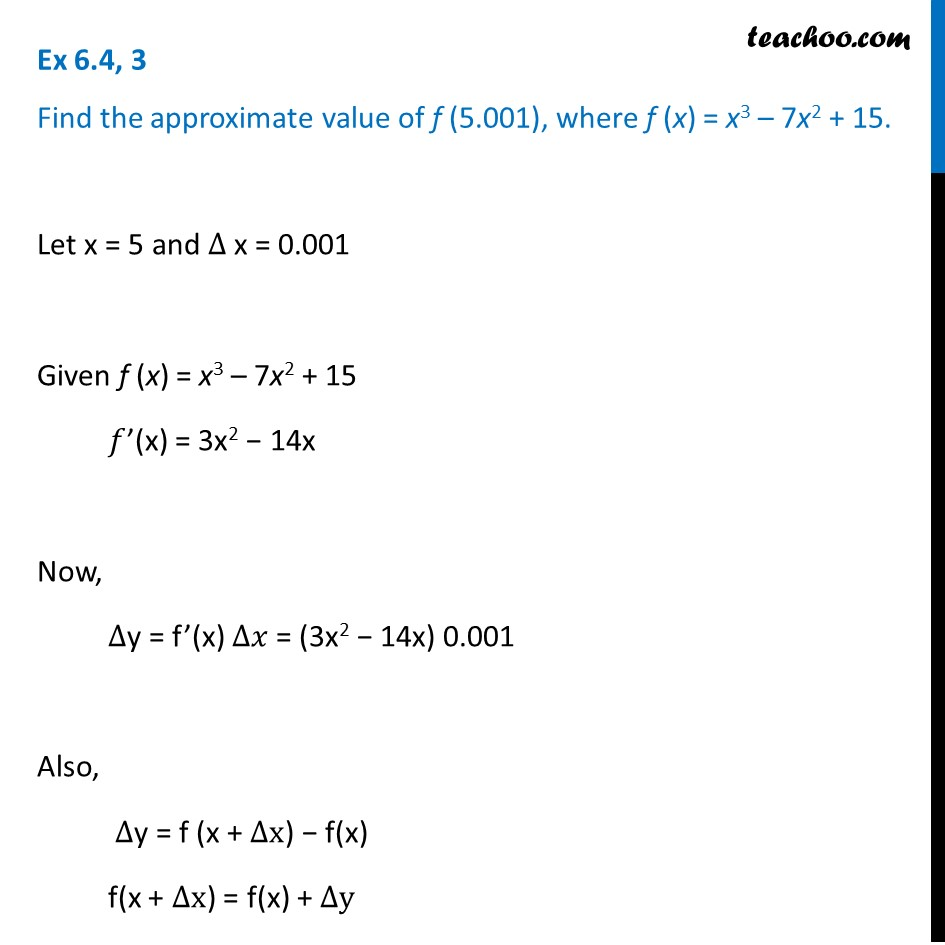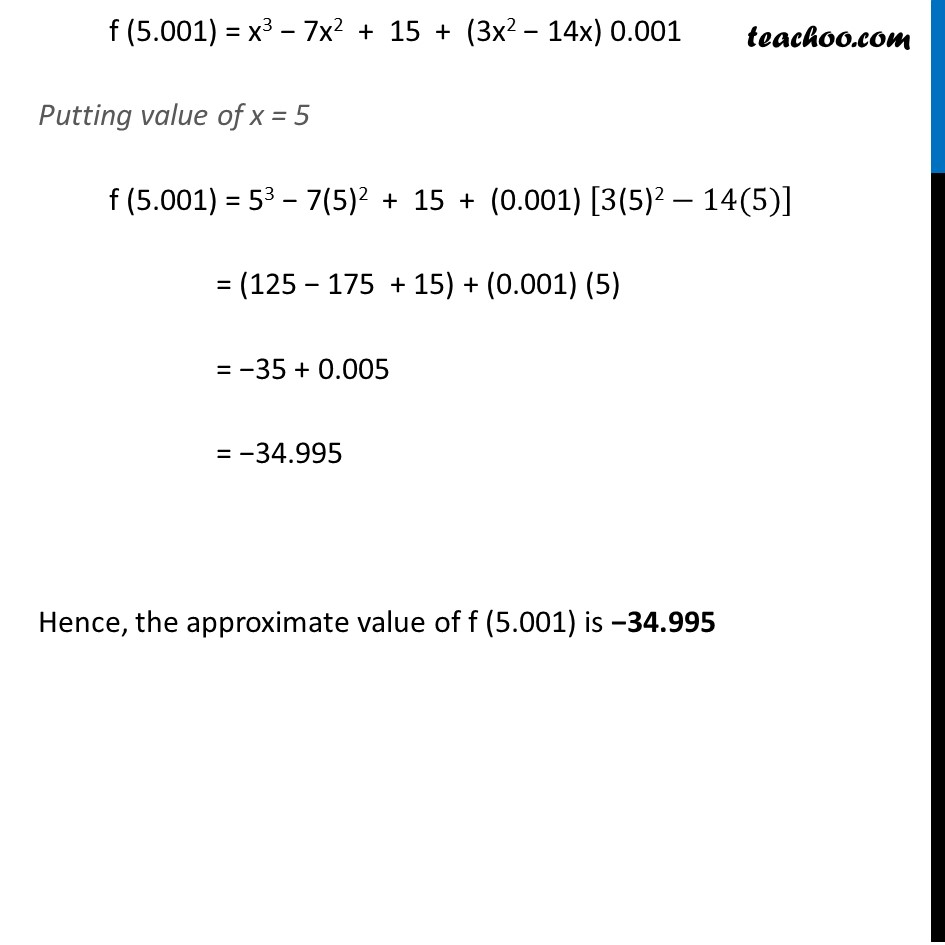Ex 6.4

Chapter 6 Class 12 Application of Derivatives
Serial order wiseGet live Maths 1-on-1 Classs - Class 6 to 12

### Transcript

Ex 6.4, 3 Find the approximate value of f (5.001), where f (x) = x3 – 7x2 + 15.Let x = 5 and ∆ x = 0.001 Given f (x) = x3 – 7x2 + 15 𝑓’(x) = 3x2 − 14x Now, ∆y = f’(x) ∆𝑥 = (3x2 − 14x) 0.001 Also, ∆y = f (x + ∆x) − f(x) f(x + ∆x) = f(x) + ∆y f (5.001) = x3 − 7x2 + 15 + (3x2 − 14x) 0.001 Putting value of x = 5 f (5.001) = 53 − 7(5)2 + 15 + (0.001) [3"(5)2" −14(5)] = (125 − 175 + 15) + (0.001) (5) = −35 + 0.005 = −34.995 Hence, the approximate value of f (5.001) is −34.995